Download Presentation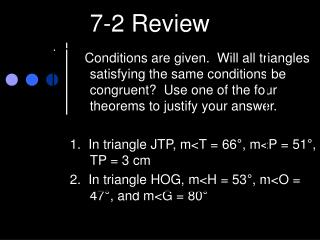7-2 Review

# 7-2 Review - PowerPoint PPT Presentation

7-2 Review. Conditions are given. Will all triangles satisfying the same conditions be congruent? Use one of the four theorems to justify your answer. 1. In triangle JTP, m<T = 66 °, m<P = 51°, TP = 3 cm 2. In triangle HOG, m<H = 53°, m<O = 47°, and m<G = 80°.I am the owner, or an agent authorized to act on behalf of the owner, of the copyrighted work described.
Download Presentation## 7-2 Review

An Image/Link below is provided (as is) to download presentation

Download Policy: Content on the Website is provided to you AS IS for your information and personal use and may not be sold / licensed / shared on other websites without getting consent from its author.While downloading, if for some reason you are not able to download a presentation, the publisher may have deleted the file from their server.

- - - - - - - - - - - - - - - - - - - - - - - - - - E N D - - - - - - - - - - - - - - - - - - - - - - - - - -
Presentation Transcript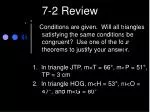### 7-2 Review

Conditions are given. Will all triangles satisfying the same conditions be congruent? Use one of the four theorems to justify your answer.

1. In triangle JTP, m<T = 66°, m<P = 51°, TP = 3 cm

2. In triangle HOG, m<H = 53°, m<O = 47°, and m<G = 80°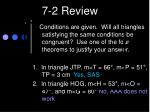### 7-2 Review

Conditions are given. Will all triangles satisfying the same conditions be congruent? Use one of the four theorems to justify your answer.

1. In triangle JTP, m<T = 66°, m<P = 51°, TP = 3 cm Yes, SAS

2. In triangle HOG, m<H = 53°, m<O = 47°, and m<G = 80° No, AAA does not workQuote of the Day

“A good hockey player plays where the puck is. A great hockey player plays where the puck is going to be.”

-Wayne Gretzky

B day Grade pages 375-377 2, 5-13, 15-18, 20-24

A day Collect Master 7-3 B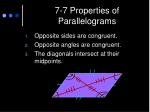7-7 Properties of Parallelograms
• Opposite sides are congruent.
• Opposite angles are congruent.
• The diagonals intersect at their midpoints.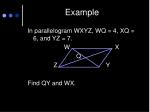Example

In parallelogram WXYZ, WQ = 4, XQ = 6, and YZ = 7.

W X

Q

Z Y

Find QY and WX.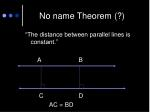No name Theorem (?)

“The distance between parallel lines is constant.”

A B

C D

AC = BD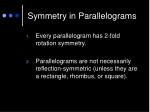Symmetry in Parallelograms
• Every parallelogram has 2-fold rotation symmetry.
• Parallelograms are not necessarily reflection-symmetric (unless they are a rectangle, rhombus, or square).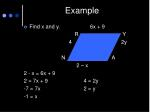Example
• Find x and y. 6x + 9

R Y

4 2y

N A

2 – x

2 - x = 6x + 9

2 = 7x + 9 4 = 2y

-7 = 7x 2 = y

-1 = x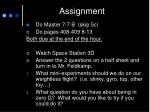Assignment
• Do Master 7-7 B (skip 5c)
• Do pages 408-409 8-13

Both due at the end of the hour.

• Watch Space Station 3D
• Answer the 2 questions on a half sheet and turn in to Mr. Feldkamp.
• What mini-experiments should we do on our weightless flight? (i.e. slinky, gyro, top, other toy…)
• What question do you have about being in zero G? What would you like to try if you could do it?